Ex 6.5

Chapter 6 Class 10 Triangles
Serial order wise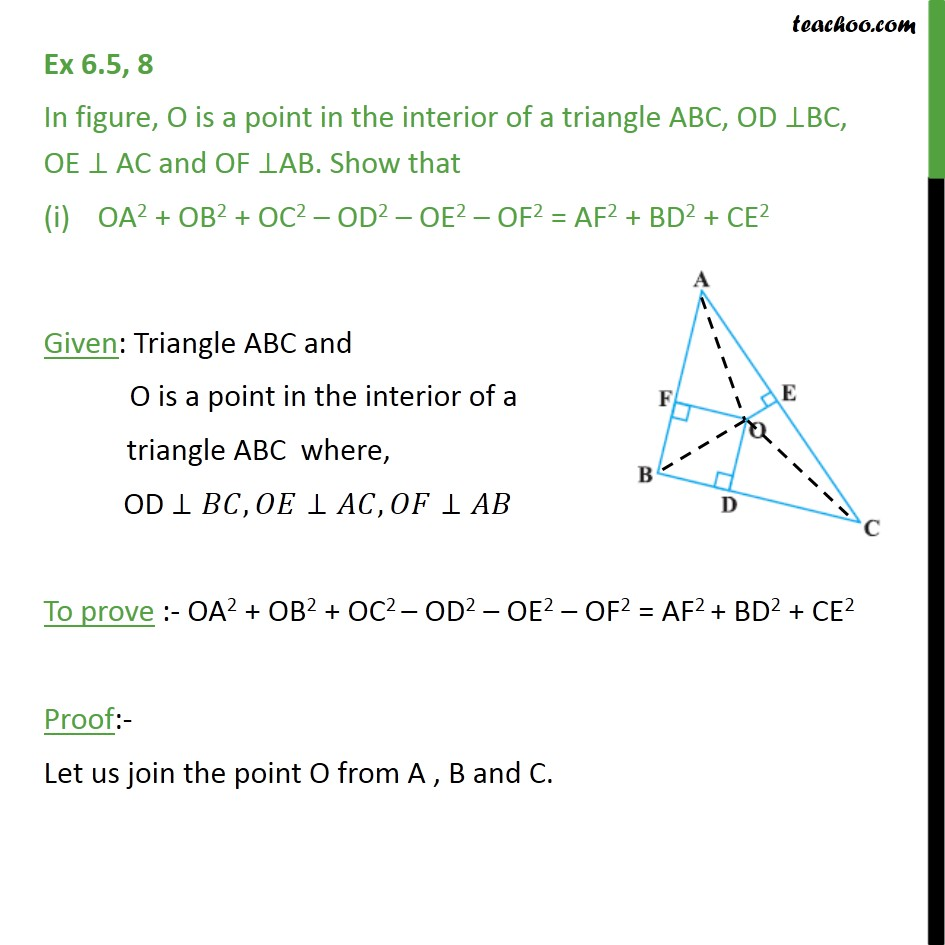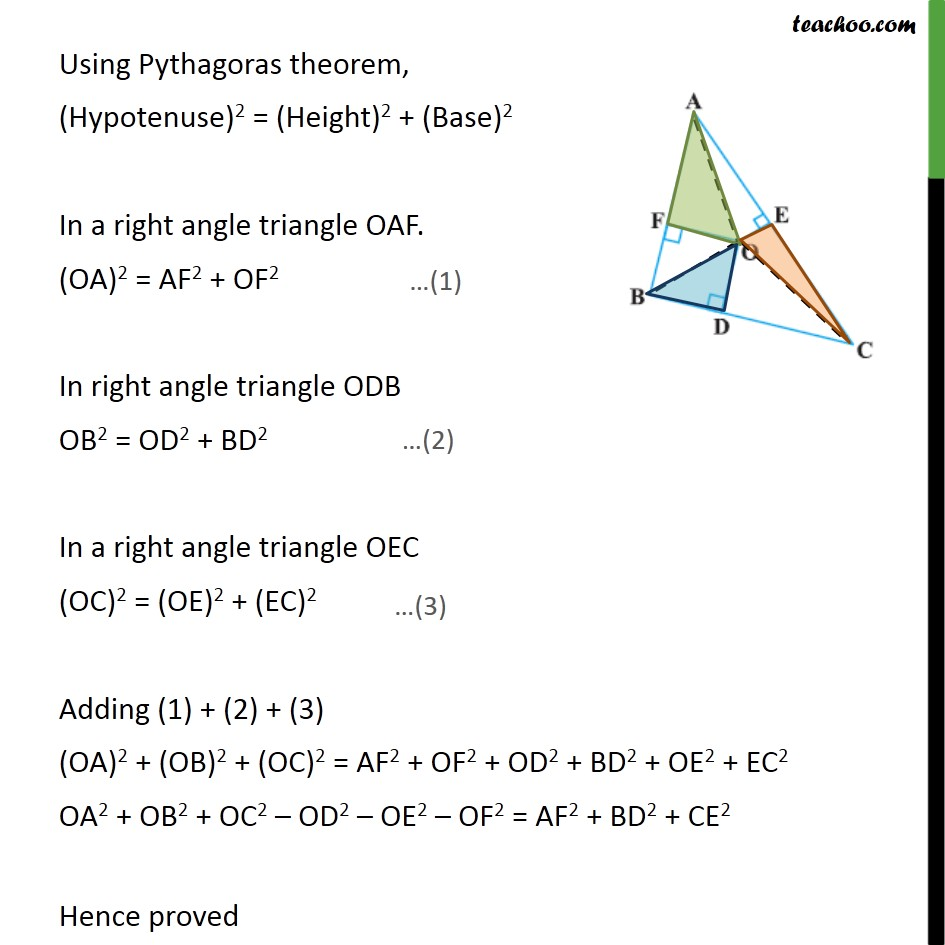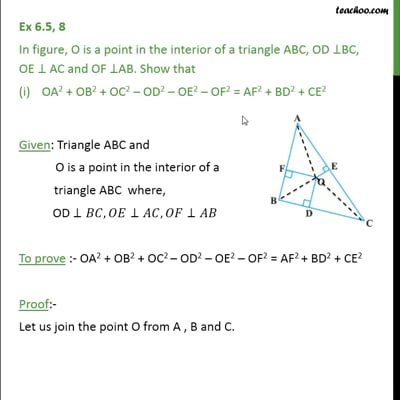This video is only available for Teachoo black users

\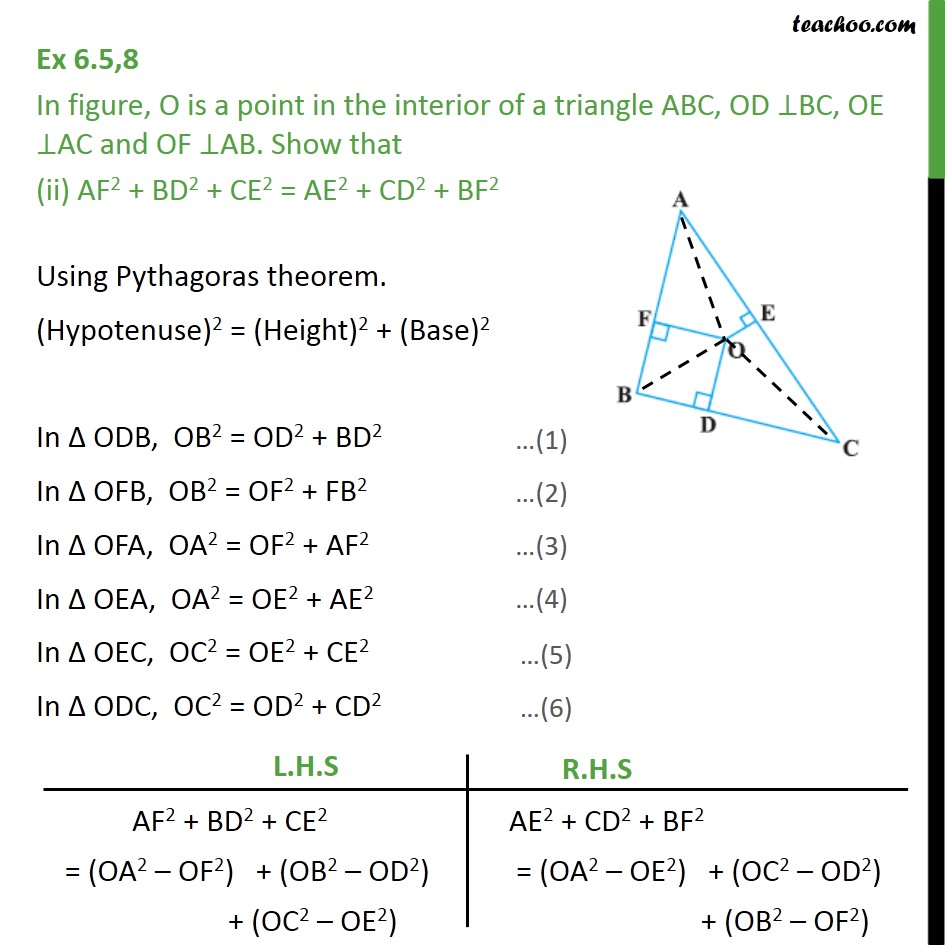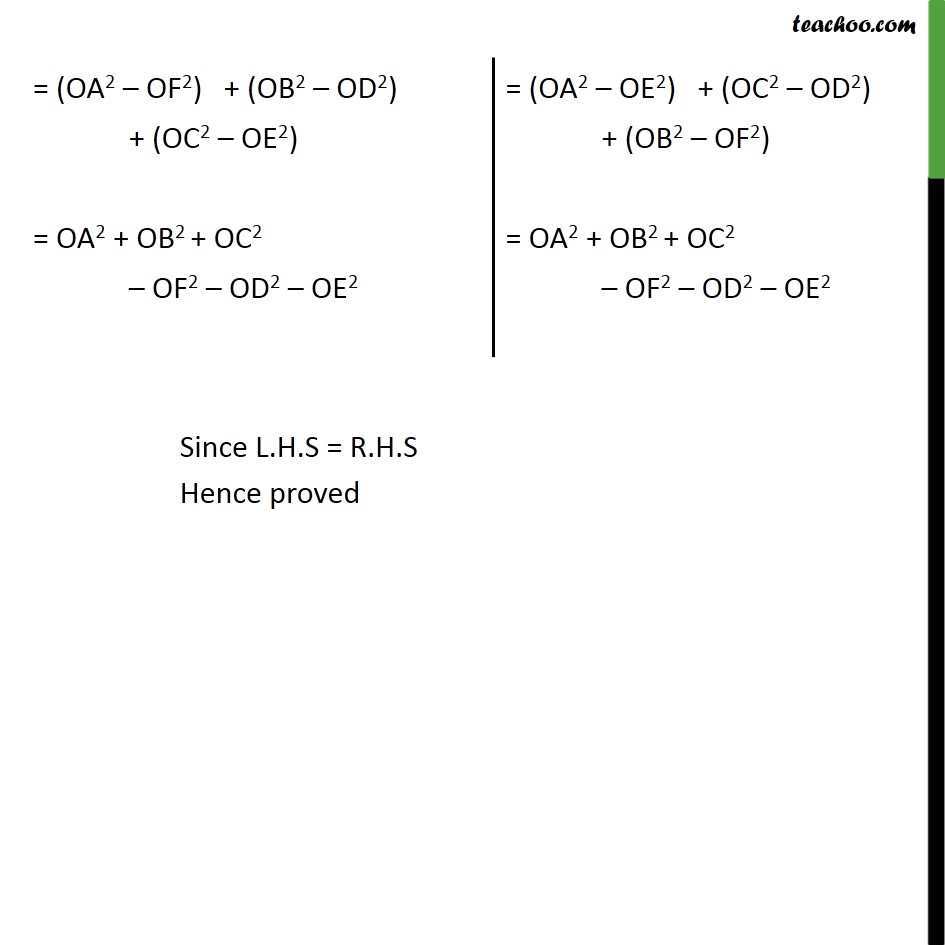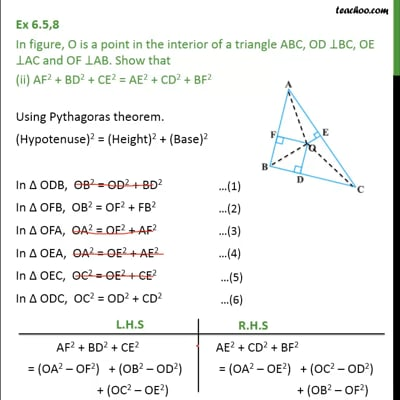This video is only available for Teachoo black users

Solve all your doubts with Teachoo Black (new monthly pack available now!)

### Transcript

Ex 6.5, 8 In figure, O is a point in the interior of a triangle ABC, OD ⊥BC, OE ⊥ AC and OF ⊥AB. Show that OA2 + OB2 + OC2 – OD2 – OE2 – OF2 = AF2 + BD2 + CE2 Given: Triangle ABC and O is a point in the interior of a triangle ABC where, OD ⊥𝐵𝐶,𝑂𝐸⊥𝐴𝐶,𝑂𝐹⊥𝐴𝐵 To prove :- OA2 + OB2 + OC2 – OD2 – OE2 – OF2 = AF2 + BD2 + CE2 Proof:- Let us join the point O from A , B and C. Using Pythagoras theorem, (Hypotenuse)2 = (Height)2 + (Base)2 In a right angle triangle OAF. (OA)2 = AF2 + OF2 In right angle triangle ODB OB2 = OD2 + BD2 In a right angle triangle OEC (OC)2 = (OE)2 + (EC)2 Adding (1) + (2) + (3) (OA)2 + (OB)2 + (OC)2 = AF2 + OF2 + OD2 + BD2 + OE2 + EC2 OA2 + OB2 + OC2 – OD2 – OE2 – OF2 = AF2 + BD2 + CE2 Hence proved Ex 6.5,8 In figure, O is a point in the interior of a triangle ABC, OD ⊥BC, OE ⊥AC and OF ⊥AB. Show that (ii) AF2 + BD2 + CE2 = AE2 + CD2 + BF2 Using Pythagoras theorem. (Hypotenuse)2 = (Height)2 + (Base)2 In Δ ODB, OB2 = OD2 + BD2 In Δ OFB, OB2 = OF2 + FB2 In Δ OFA, OA2 = OF2 + AF2 In Δ OEA, OA2 = OE2 + AE2 In Δ OEC, OC2 = OE2 + CE2 In Δ ODC, OC2 = OD2 + CD2